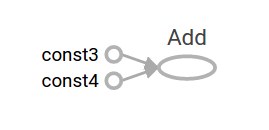Watch talks from the 2019 TensorFlow Dev Summit

# 简介

• 如果您能够直接使用低阶 TensorFlow 操作，实验和调试都会更直接。
• 在使用更高阶的 API 时，能够理解其内部工作原理。

## 设置

• 如何使用 Python 编程。
• 对阵列有所了解。
• 理想情况下，最好对机器学习有一定的了解。

from __future__ import absolute_import
from __future__ import division
from __future__ import print_function

import numpy as np
import tensorflow as tf


## 张量值

TensorFlow 中的核心数据单位是张量。一个张量由一组形成阵列（任意维数）的原始值组成。张量的是它的维数，而它的形状是一个整数元组，指定了阵列每个维度的长度。以下是张量值的一些示例：

3. # a rank 0 tensor; a scalar with shape [],
[1., 2., 3.] # a rank 1 tensor; a vector with shape 
[[1., 2., 3.], [4., 5., 6.]] # a rank 2 tensor; a matrix with shape [2, 3]
[[[1., 2., 3.]], [[7., 8., 9.]]] # a rank 3 tensor with shape [2, 1, 3]


TensorFlow 使用 numpy 阵列来表示张量

## TensorFlow Core 演示

1. 构建计算图 (tf.Graph)。
2. 运行计算图（使用 tf.Session）。

### 图

• 操作（简称“op”）：图的节点。操作描述了消耗和生成张量的计算。
• 张量：图的边。它们代表将流经图的值。大多数 TensorFlow 函数会返回 tf.Tensors

a = tf.constant(3.0, dtype=tf.float32)
b = tf.constant(4.0) # also tf.float32 implicitly
total = a + b
print(a)
print(b)
print(total)


Tensor("Const:0", shape=(), dtype=float32)
Tensor("Const_1:0", shape=(), dtype=float32)


### TensorBoard

TensorFlow 提供了一个名为 TensorBoard 的实用程序。TensorBoard 的诸多功能之一是将计算图可视化。您只需要使用几个简单的命令就能轻松完成此操作。

writer = tf.summary.FileWriter('.')


events.out.tfevents.{timestamp}.{hostname}


tensorboard --logdir .### 会话 (Session)

sess = tf.Session()
print(sess.run(total))


7.0


print(sess.run({'ab':(a, b), 'total':total}))


{'total': 7.0, 'ab': (3.0, 4.0)}


vec = tf.random_uniform(shape=(3,))
out1 = vec + 1
out2 = vec + 2
print(sess.run(vec))
print(sess.run(vec))
print(sess.run((out1, out2)))


[ 0.52917576  0.64076328  0.68353939]
[ 0.66192627  0.89126778  0.06254101]
(
array([ 1.88408756,  1.87149239,  1.84057522], dtype=float32),
array([ 2.88408756,  2.87149239,  2.84057522], dtype=float32)
)


### 供给

x = tf.placeholder(tf.float32)
y = tf.placeholder(tf.float32)
z = x + y


print(sess.run(z, feed_dict={x: 3, y: 4.5}))
print(sess.run(z, feed_dict={x: [1, 3], y: [2, 4]}))


7.5
[ 3.  7.]


## 数据集

my_data = [
[0, 1,],
[2, 3,],
[4, 5,],
[6, 7,],
]
slices = tf.data.Dataset.from_tensor_slices(my_data)
next_item = slices.make_one_shot_iterator().get_next()


while True:
try:
print(sess.run(next_item))
except tf.errors.OutOfRangeError:
break


r = tf.random_normal([10,3])
dataset = tf.data.Dataset.from_tensor_slices(r)
iterator = dataset.make_initializable_iterator()
next_row = iterator.get_next()

sess.run(iterator.initializer)
while True:
try:
print(sess.run(next_row))
except tf.errors.OutOfRangeError:
break


## 层

### 创建层

x = tf.placeholder(tf.float32, shape=[None, 3])
linear_model = tf.layers.Dense(units=1)
y = linear_model(x)


### 初始化层

init = tf.global_variables_initializer()
sess.run(init)


### 执行层

print(sess.run(y, {x: [[1, 2, 3],[4, 5, 6]]}))


[[-3.41378999]
[-9.14999008]]


### 层函数的快捷方式

x = tf.placeholder(tf.float32, shape=[None, 3])
y = tf.layers.dense(x, units=1)

init = tf.global_variables_initializer()
sess.run(init)

print(sess.run(y, {x: [[1, 2, 3], [4, 5, 6]]}))


## 特征列

features = {
'sales' : [, , , ],
'department': ['sports', 'sports', 'gardening', 'gardening']}

department_column = tf.feature_column.categorical_column_with_vocabulary_list(
'department', ['sports', 'gardening'])
department_column = tf.feature_column.indicator_column(department_column)

columns = [
tf.feature_column.numeric_column('sales'),
department_column
]

inputs = tf.feature_column.input_layer(features, columns)


var_init = tf.global_variables_initializer()
table_init = tf.tables_initializer()
sess = tf.Session()
sess.run((var_init, table_init))


print(sess.run(inputs))


[[  1.   0.   5.]
[  1.   0.  10.]
[  0.   1.   8.]
[  0.   1.   9.]]


## 训练

### 定义数据

x = tf.constant([, , , ], dtype=tf.float32)
y_true = tf.constant([, [-1], [-2], [-3]], dtype=tf.float32)


### 定义模型

linear_model = tf.layers.Dense(units=1)

y_pred = linear_model(x)


sess = tf.Session()
init = tf.global_variables_initializer()
sess.run(init)

print(sess.run(y_pred))


[[ 0.02631879]
[ 0.05263758]
[ 0.07895637]
[ 0.10527515]]


### 损失

loss = tf.losses.mean_squared_error(labels=y_true, predictions=y_pred)

print(sess.run(loss))


2.23962


### 训练

TensorFlow 提供了执行标准优化算法的优化器。这些优化器被实现为 tf.train.Optimizer 的子类。它们会逐渐改变每个变量，以便将损失最小化。最简单的优化算法是梯度下降法，由 tf.train.GradientDescentOptimizer 实现。它会根据损失相对于变量的导数大小来修改各个变量。例如：

optimizer = tf.train.GradientDescentOptimizer(0.01)
train = optimizer.minimize(loss)


for i in range(100):
_, loss_value = sess.run((train, loss))
print(loss_value)


1.35659
1.00412
0.759167
0.588829
0.470264
0.387626
0.329918
0.289511
0.261112
0.241046
...


### 完整程序

x = tf.constant([, , , ], dtype=tf.float32)
y_true = tf.constant([, [-1], [-2], [-3]], dtype=tf.float32)

linear_model = tf.layers.Dense(units=1)

y_pred = linear_model(x)
loss = tf.losses.mean_squared_error(labels=y_true, predictions=y_pred)

train = optimizer.minimize(loss)

init = tf.global_variables_initializer()

sess = tf.Session()
sess.run(init)
for i in range(100):
_, loss_value = sess.run((train, loss))
print(loss_value)

print(sess.run(y_pred))


## 后续步骤

• 自定义 Estimator，了解如何使用 TensorFlow 构建自定义模型。掌握 TensorFlow Core 知识有助于理解和调试您自己的模型。